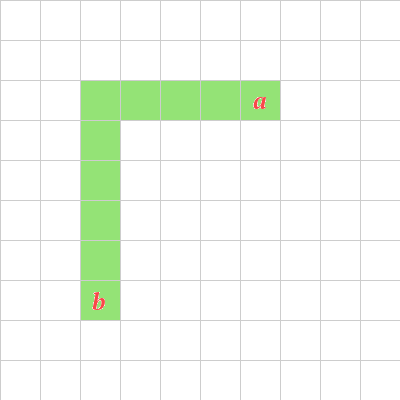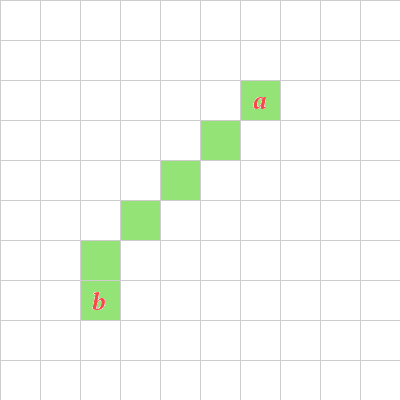# Distance Measures Redux -- Unifying and Tweaking

Posted on August 29, 2016
Tags: geometry

After talking about distance calculations the other day, I was thinking about how we could generalize the measures.

Looking back on the D&D distance calculation, I think it can encompass civ and Manhattan distances, too.The shortest taxi drive

First, for simplicity’s sake, I’m going to refer to $$x_d$$ instead of $$|x_1 - x_2|$$, and likewise for $$y_d$$. With that convenience, I’ll contract it to a single line rather than spreading it over three. Finally, I’m going to discard the floor conversion ($$\lfloor \rfloor$$) for the moment.

$d = \max(x_d, y_d) - \min(x_d, y_d) + 1.5 \times (\min(x_d, y_d))$

Note that “1.5”. I think that’s the trick. If we manipulate that, we should be able to replicate the other, simpler calculations.

Remember that the 1.5 represents the cost of making a diagonal rather than a cardinal move. Since taxis can only move cardinally, they take two steps to move diagonally. Let’s plug “2” into that and see what happens:

$d = \max(x_d, y_d) - \min(x_d, y_d) + 2 \times (\min(x_d, y_d))$

The $$\min$$ cancels out a bit:

$d = \max(x_d, y_d) + 1 \times (\min(x_d, y_d))$

Which further simplifies:

$d = x_d + y_d$

Which is the Manhattan formula. Success!The shortest discrete drive

Let’s try it for the Civ distance. Where taxis take 2 moves to go diagonally, civilizations do it in 1:

$d = \max(x_d, y_d) - \min(x_d, y_d) + 1 \times (\min(x_d, y_d))$

Those $$\min$$’s cancel out completely:

d = (x_d, y_d)

… which is, again, exactly how we calculate Civ distance. Excellent, well done!

That leaves us with the general formula:

$d = \max(x_d, y_d) - \min(x_d, y_d) + k \times (\min(x_d, y_d))$

Where $$k$$ is the diagonal cost.

### TweakingRemember that the D&D distance ended up being closest to Euclidean. If $$x_d$$ and $$y_d$$ are equal – that is, if we’re going at exactly a 45-degree angle – the Euclidean distance is $$x_d \times \sqrt(2)$$. That $$sqrt(2)$$ is 1.41421….

Last post, I mused that, since 1.5 was much closer to $$sqrt(2)$$ than either 1 (civ) or 2 (taxi), that is why D&D distance was such a good Euclidean approximation.

But, could it be better? If we plug $$k=1.4$$ into our general distance equation, does it come closer?

Well, so I wrote a script to test this. It’s up in the same repo as last time.

It generates a set of random points, then calculates the Euclidean distance from $$(0,0)$$ to each point. For each of the other measures, it calculates the same distance, and compares it to the Euclidean, keeping the difference. At the end, it prints out the total difference for each measure.It looks a bit like this:

\$ ./test_distances.py
taxi  diff: 12848
civ   diff: 5146
dandd diff: 3851
other diff: 2071

As you can see, the “other” calculation ($$k = 1.4$$) has the least difference from the Euclidean distance, and so is a nice, quite accurate measure.

1.4 is a tricky number. If you wanted to use it in a game of some sort, though, you could cost it out differently – the thing that matters is the ratio. For example, it might cost 5 action points to move in a cardinal direction, but 7 action points to move diagonally. That would be quite true to Euclid, while nicely operating in our discrete movement world.

Thanks for reading through, and hope it was interesting for you, too.

I'd love to have a conversation, but I'm not interested in having a comments section. Instead, ping me on twitter or some other media. I'll try to link to responses from the relevant post page.